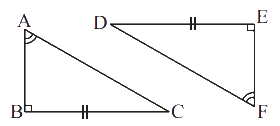# Ex.7.2 Q10 Congruence of Triangles - NCERT Maths Class 7

Go back to  'Ex.7.2'

## Question

Explain, why$$ΔABC ≅ ΔFED$$.

Video Solution
Congruence Of Triangles
Ex 7.2 | Question 10

## Text Solution

What is known?

Two right angles triangles $$ΔABC$$ and $$ΔFED$$ in which one side and one angle are equal.

What is unknown?

Why $$ΔABC ≅ ΔFED$$?

Reasoning:

This question is based on congruence of a right-angled triangle. In this question, one angle and a side of a right-angled triangle are equal to the corresponding one angle and a side of another right-angled triangle. Now, by using congruence based on right-angled triangle, you can find the reason why these two triangles are congruent.

Steps:

Given,

In triangles, $$ABC$$ and $$FED$$,

\begin{align}\angle \text{A}\,&=\ \angle \,\text{F}\\ \angle \text{B}\,&=\,\,\angle \text{E=9}{{\text{0}}^{\circ }}\\\rm{} BC &= ED\end{align}

Therefore, $$ΔABC ≅ ΔFED$$ (By $$RHS$$ congruence rule)

Learn from the best math teachers and top your exams

• Live one on one classroom and doubt clearing
• Practice worksheets in and after class for conceptual clarity
• Personalized curriculum to keep up with school